# From spss to R, part 3

In this post we will start with a build-in dataset and some basic ggplot graphics. In the next post we will combine dplyr and ggplot to do awesome stuff with the Dutch University student counts from the previous lessons.

We will work with the build-in dataset mtcars.

There are many datasets in r library(help = "datasets") but in many examples online you will see the iris and mtcars examples. Find more information about the dataset with ?iris. As you can see it’s possible to include metadata about datasets in a package, so in the future you might want to share your data in the form of a package with all your code included.

But first back to the mtcars dataset and then to graphing (is that a word?).

## The mtcars dataset

According to ?mtcars: “The data was extracted from the 1974 Motor Trend US magazine, and comprises fuel consumption and 10 aspects of automobile design and performance for 32 automobiles (1973–74 models).”

The mtcars documentation gives us an example of displaying the mtcars information:

pairs(mtcars, main = "mtcars data")
# this is one plot next is an other
coplot(mpg ~ disp | as.factor(cyl), data = mtcars,
panel = panel.smooth, rows = 1)


## Plotting graphs in R

R has three plotting systems, each based on different ideas. The base plot system is very fast and is very useful for quick views of your data. But you need to tinker a lot to make plots nicer. Then there is the lattice system 1. Which is probably very useful, but I mostly use ggplot. For you ggplot might be THE reason to come to R.

To make the most of ggplot, and not go insane, you will need to understand the underlying principles. the gg in ggplot stand for the grammar of graphics. A concept based on the work of Wilkinson and Wills 2, and put into a graphics package by Hadley Wickham 3 (this is quite a good read). The most important thing to realize is this: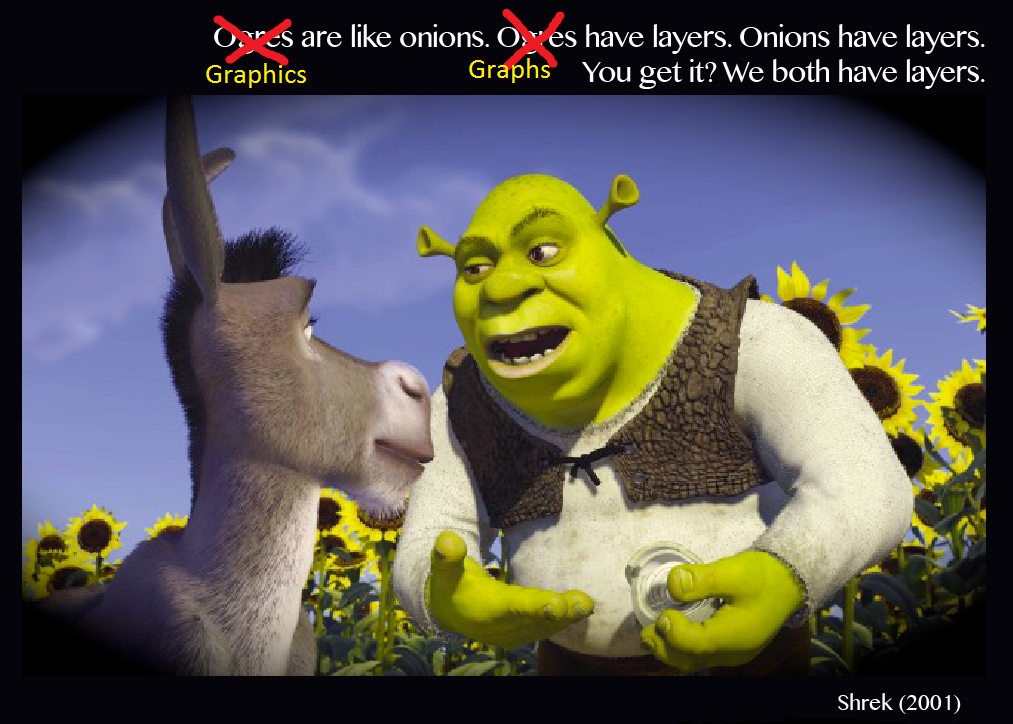Every graph consists of the basic data, some transformations to axes, title information and the representation of the data. In ggplot you have to specify the data, and the aesthetics (some mapping of the position of x, and y, colours and shapes). Furthermore you have to tell ggplot what type of chart you want. Bars, lines, and points are all examples of geometric objects or geoms for short.

## Example: a basic scatter plot in ggplot

library(ggplot2)
str(mtcars) # just to show you the variables in mtcars.
ggplot(data = mtcars, aes(x= mpg, y= wt) ) + geom_point()


Which will look like this: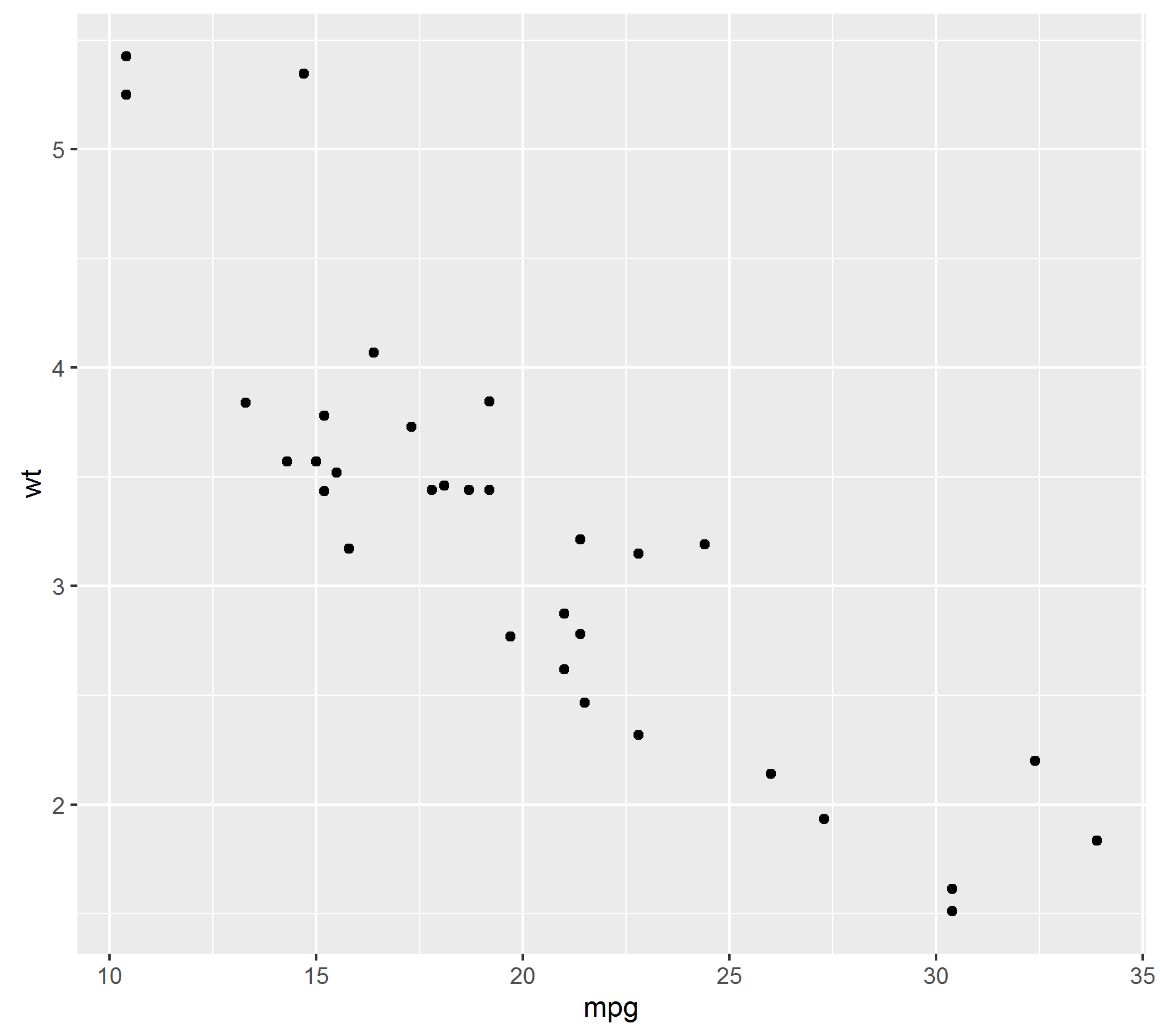We called a ggplot element, specified the data, then the aesthetics so that miles per gallon (mpg) is on the x axis and weight (wt) on the Y axis.

• select the first part ggplot(data = mtcars, aes(x= mpg, y= wt) ) and execute ctrl/cmd-r
• what happens?

We need to tell ggplot what kind of layer to put on top. In this case we’ve added points. Also note the + sign. In dplyr we used a pipe (%>%) operator to link stuff together, within ggplot we literally add layers together with the + sign.

ggplot(data = mtcars, aes(x= mpg, y= wt )) + geom_point( color = "red" )


We’ve specified a color in the point layer. But much more effective is to use color as an extra dimension in your plots.

ggplot(data = mtcars, aes(mpg,  wt )) + geom_point(aes(color = as.factor(gear)))
# note that we don't have to tell arguments in functions what they are.
# according to ?ggplot    :
# ggplot(data = NULL, mapping = aes(), ..., environment = parent.frame())
# the first argument is data, then follows the mapping with aes
# ?aes
# aes(x, y, ...)
# and the first argument of aes is always x and the second is y.
# you CAN change the order of arguments, but then you WILL need to
# explicitly call the arguments:
ggplot(aes(y=wt, x=mpg ), data = mtcars, ) + geom_point(aes(color = as.factor(gear)))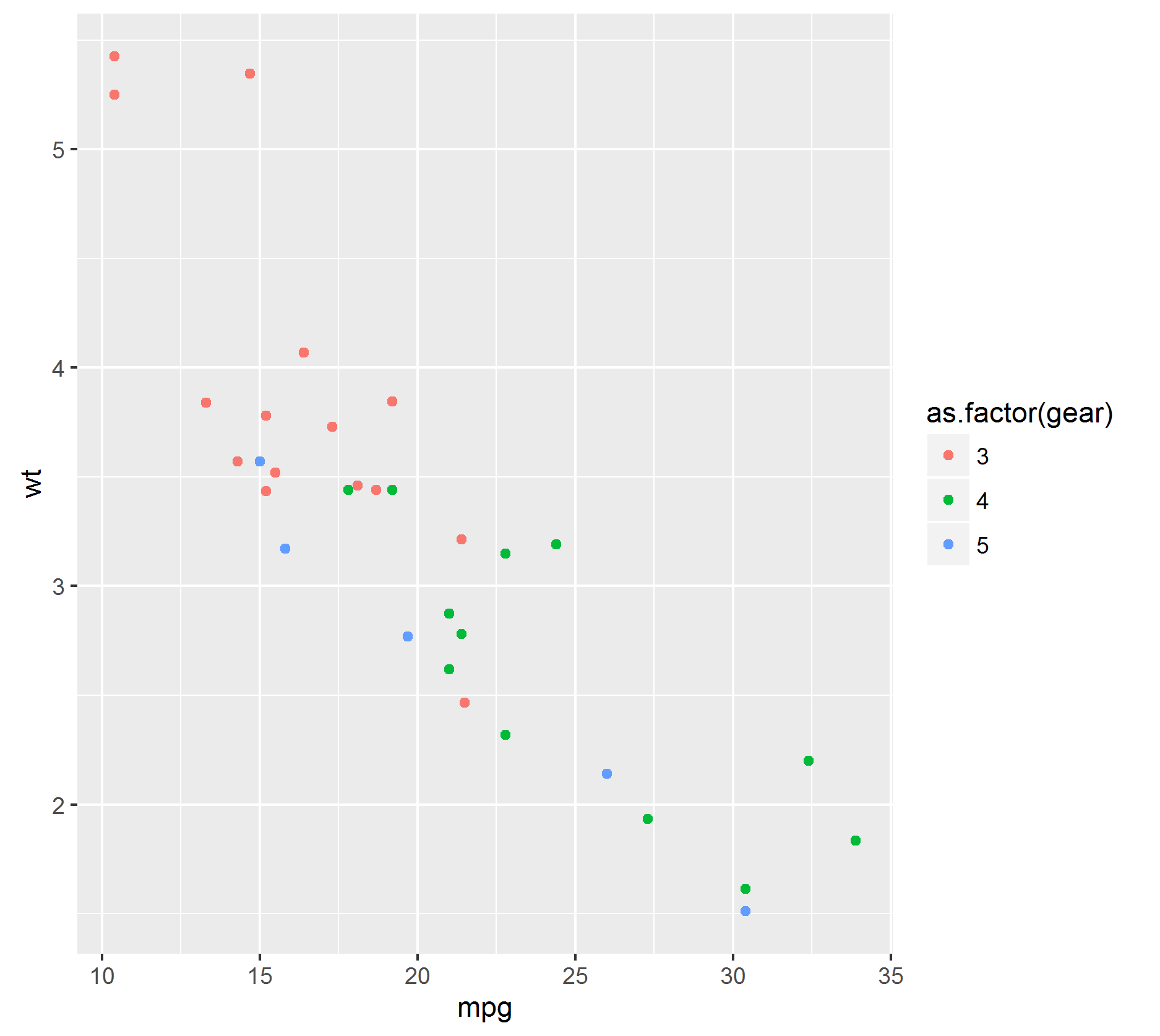• Did you check that lines 1 and 11 had the same output?

Now I told ggplot that gear was a factor. If your dataset is clean and nicely formatted you don’t have to do this. However this dataset has only numeric values, while some columns are actually factors.

• repeat the call from line 1 but change the geom_point with: geom_point(aes(color = gear))
• What did just happen?

For numeric variables, ggplot takes one color and changes the gradient from lowest to highest level of that variable. The colors for factor variables are maximaly distinct so you can identify groups easier.

• Try the following code ggplot(data = mtcars, aes(mpg, wt )) + geom_point(color = gear) and compare with: ggplot(data = mtcars, aes(mpg, wt )) + geom_point(aes(color = gear))

• What happened? Why didn’t the first work?

• but this one does:

• ggplot(data = mtcars, aes(x= mpg, y= wt )) + geom_point( color = "red" )

You will have to think about the aes() command as something that depends on you data. A way to transform the variables from your data into graphic elements. But if you want to add a color or change the size of things that do not depend on your data, you must tell it seperately to the geom.

So I said layers. let’s add layers.

ggplot(data = mtcars, aes(x= mpg, y= wt)) +
geom_point(aes( color = as.factor(gear) )) +
geom_smooth(method = lm) # a linear model Y ~ x smoothing


In this example there are three layers, a base layer, points and a smoothing on top of the points. As you add layers, each layer has their elements specified by you or inherits them from the base element ggplot(). In the above example geom_smooth() inherits the part [ aes(x, y) ] from ggplot(data = mtcars, aes(x= mpg, y= wt)).

ggplot()+geom_point(data = mtcars, aes(x= mpg, y= wt)) +geom_smooth(method = lm)


This doesn’t work, because the smoothing geom doesn’t know what data or aes to use. But if we specify them, it will work.

ggplot()+geom_point(data = mtcars, aes(x= mpg, y= wt)) +
geom_smooth(data = mtcars, aes(x= mpg, y= wt), method = lm)


We will work through a few more examples, but see Further reading for more examples of graph types and resources with lists and lists of plots and how to make them.

## Example 2: a bargraph

A bargraph has only one aesthetic mapping: ggplot(data = mtcars, aes(as.factor(cyl))) + geom_bar(stat ="count")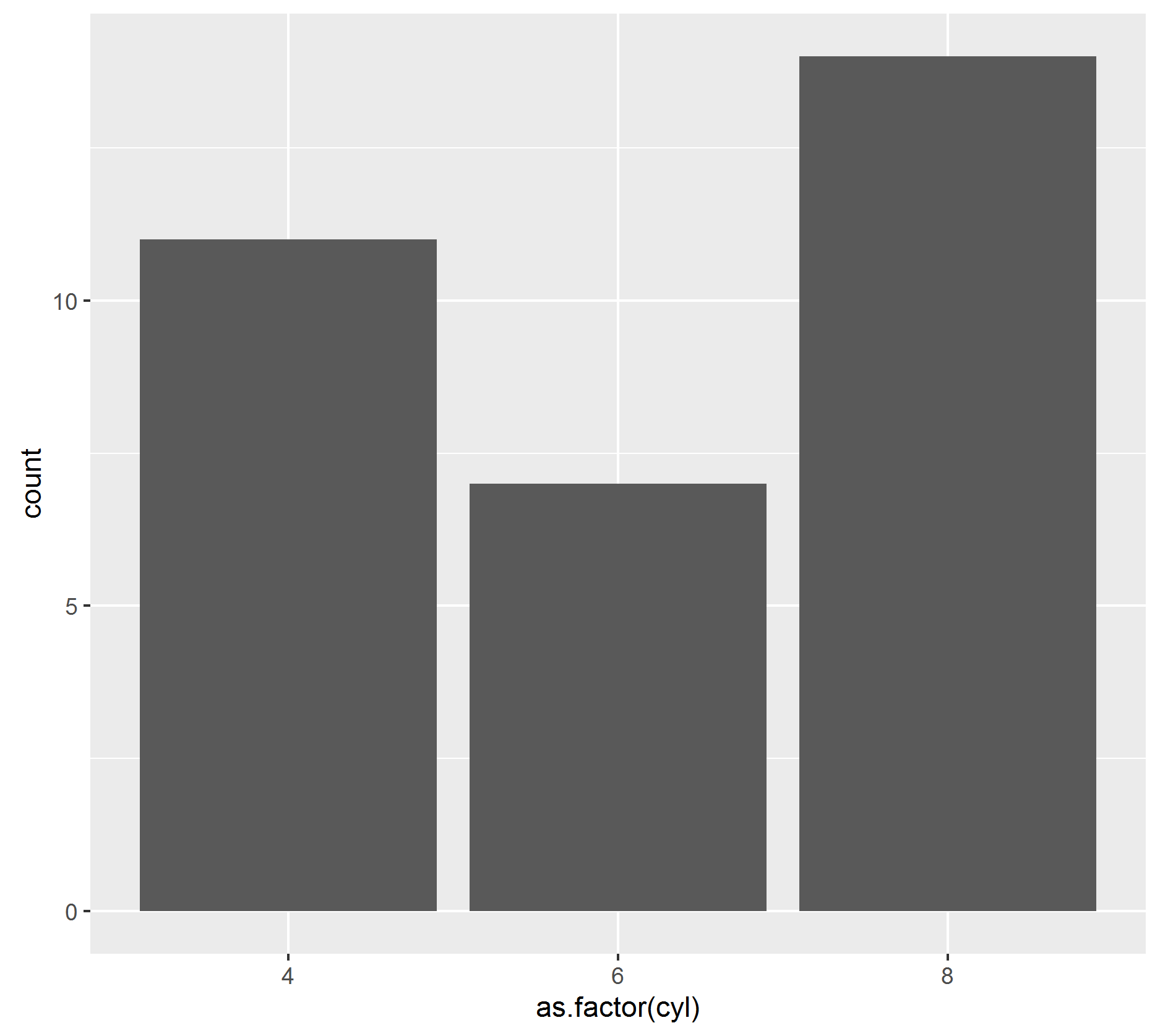only the x aesthetic is specified, the geom is a bar (see ?geom_bar) and we specified that it should perform a statistic on the data. In this case it just counts the number of occurrences. But if your data is already in frequency you could use geom_bar(stat = “identity”). For example

dat<-data.frame(
Name = c("hork", "dork", "bork"),
Frequency = c(5, 8,12)
) # a very silly dataframe
ggplot(dat, aes(Name, Frequency)) + geom_bar(stat ="identity")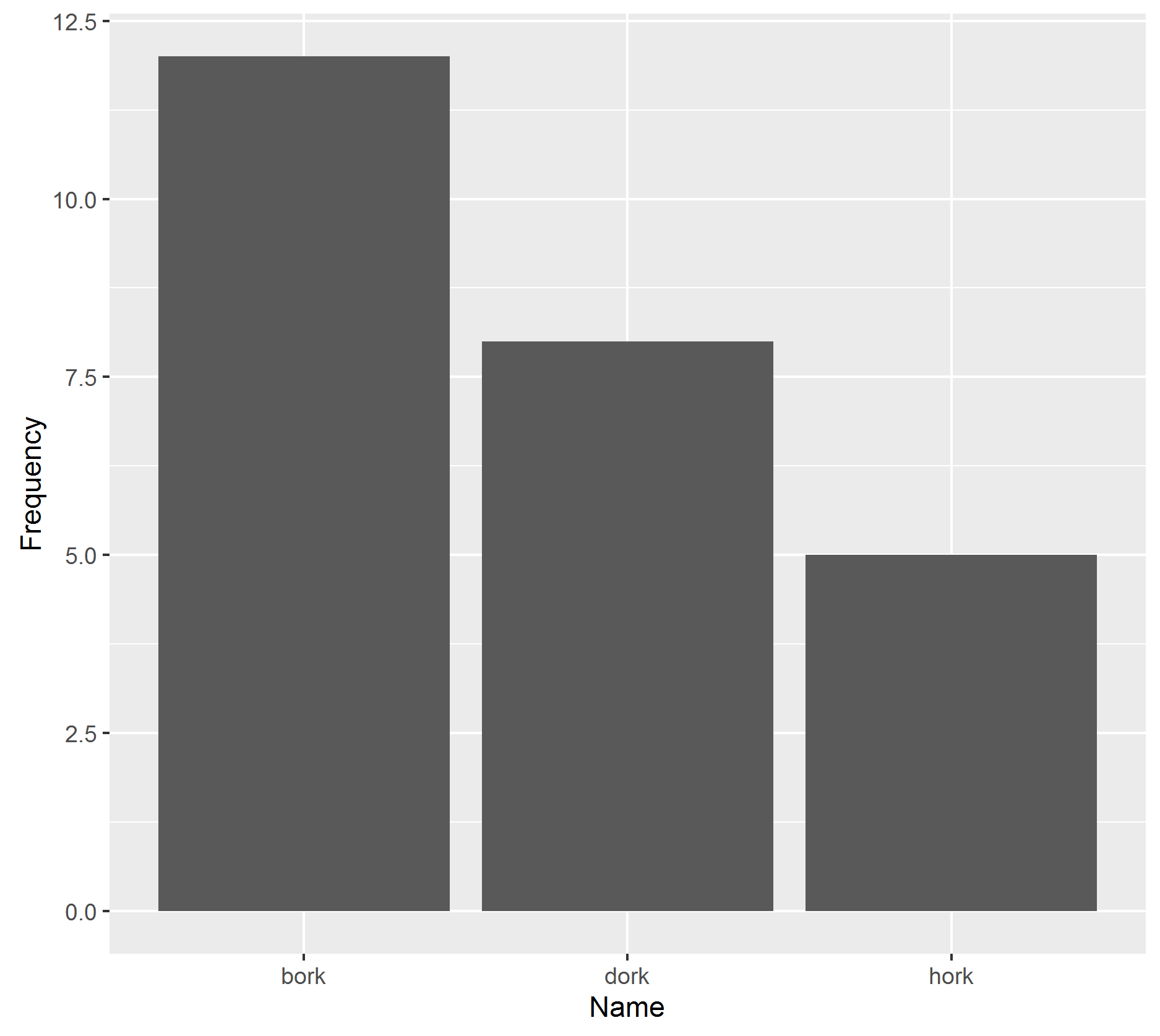## Example 3: boxplots, violingplots with points behind

Now a violinplot. The chickwts dataset is a small dataset of weights of chicks (baby chickens, not human ladies), with the diet they received.
By now you now the drill: the data is chickwts, the aes are feed and weight. Then we add a boxplot geom. See ?geom_boxplot for more info about changing parameters. I have displayed a violin plot, which displays more information about the underlying distribution.

str(chickwts)
ggplot(chickwts, aes(feed, weight)) + geom_boxplot()
ggplot(chickwts, aes(feed, weight)) + geom_violin()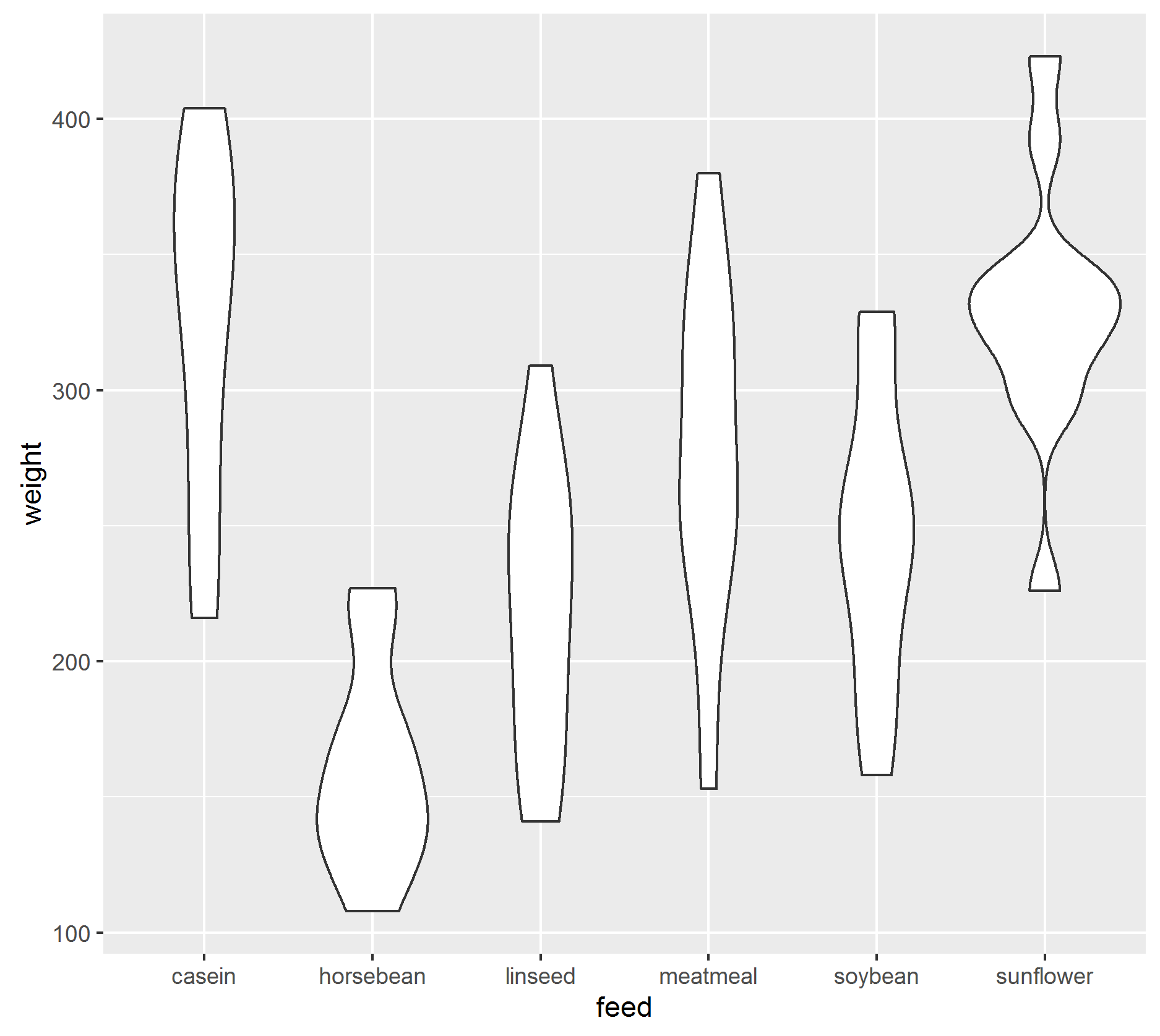Boxplots are nice, but you lose information about the middle of the distribution. So let’s plot the real distribution. ggplot(chickwts, aes(feed, weight)) + geom_point() This doesn’t really help us, what about all the chicks with the same weight and same feed? The jitter geom helps you out. ggplot(chickwts, aes(feed, weight)) + geom_jitter()

The jitter function adds some noise to points to separate the individual points. let’s combine and see what looks best

ggplot(chickwts, aes(feed, weight)) + geom_boxplot() + geom_jitter()
ggplot(chickwts, aes(feed, weight)) + geom_boxplot() + geom_point()
ggplot(chickwts, aes(feed, weight)) + geom_violin() + geom_jitter()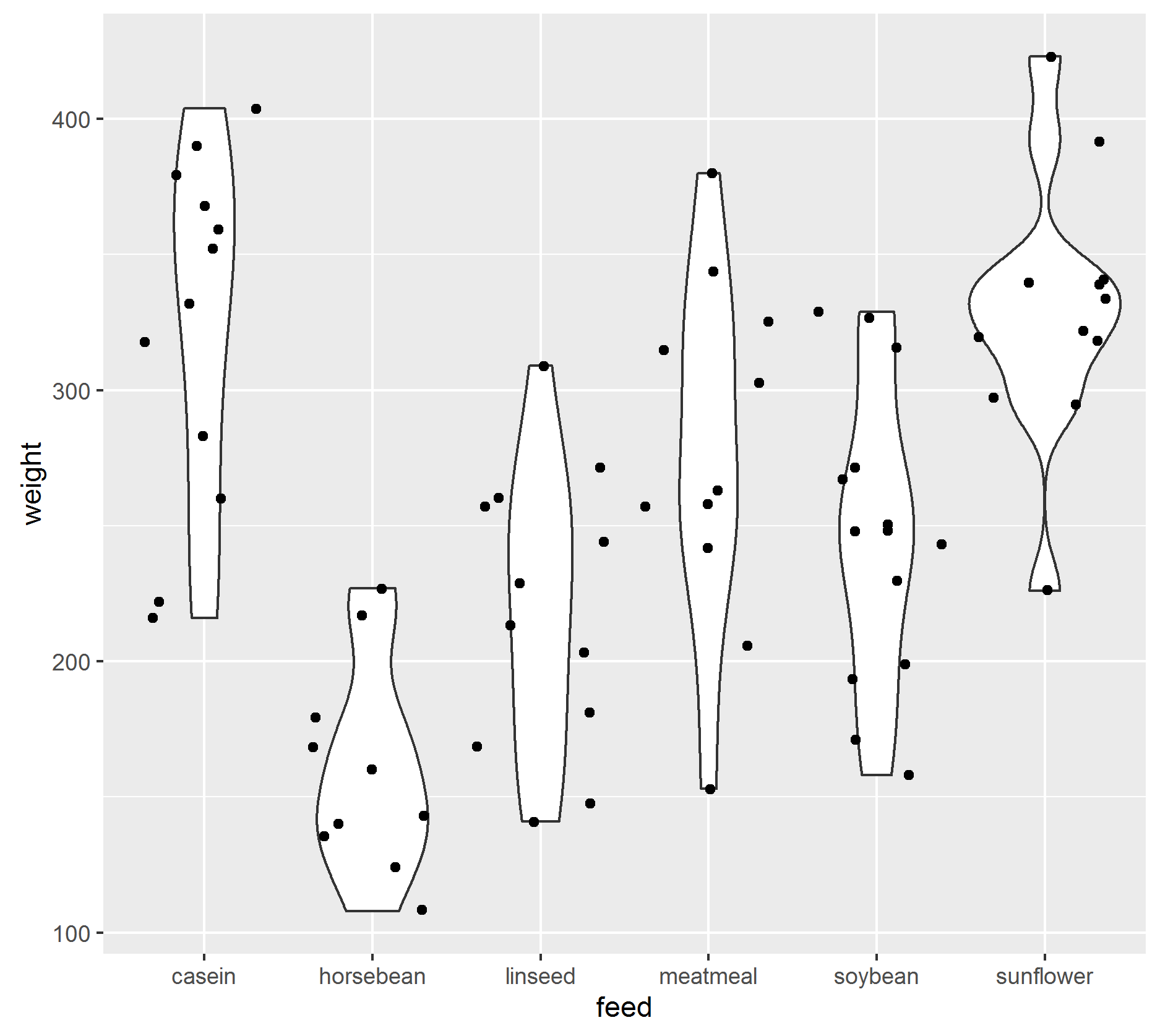I like the last one best, but for different data different visualizations are better.

ggplot(mtcars, aes(as.factor(cyl), mpg)) +geom_boxplot() +geom_point()
ggplot(mtcars, aes(as.factor(cyl), mpg)) +geom_violin() +geom_jitter( aes(color = as.factor(am)))


## Customization

Most simple plots in ggplot2 look quite good from the start, it’s a credit to Hadley Wickham that he chose ggplot2’s defaults so well. However, there are many ways to custimize your plots. Every element can change colour4, shape, etc. But if you like to change things in multiple plots you should try to change the theme. Themes change multiple things at once. For instance:

g <- ggplot(mtcars, aes(as.factor(cyl), mpg)) +geom_violin() +geom_jitter( aes(color = as.factor(am)))
# I saved the entire thing to a variable.
# if you select the g and press ctrl/cmd r it will display the plot again.
# even better the saved object behaves just as before. We can add things to it.
# try the following things
g + theme_bw()
g + theme_dark()  # the addition doesn't stick untill you save it to a variable
g + theme_void()


It is relatively easy to change parts of themes, or to create a whole new theme. But quite easy is also the ggtheme package, see their website .

install.packages("ggthemes")
library(ggthemes)
g + theme_wsj()
g + theme_tufte()  # very clean theme based on edward tufte 's ideas about graphs
g + theme_base()
g + theme_excel()  # you will love this, especially the description
g + theme_fivethirtyeight()
`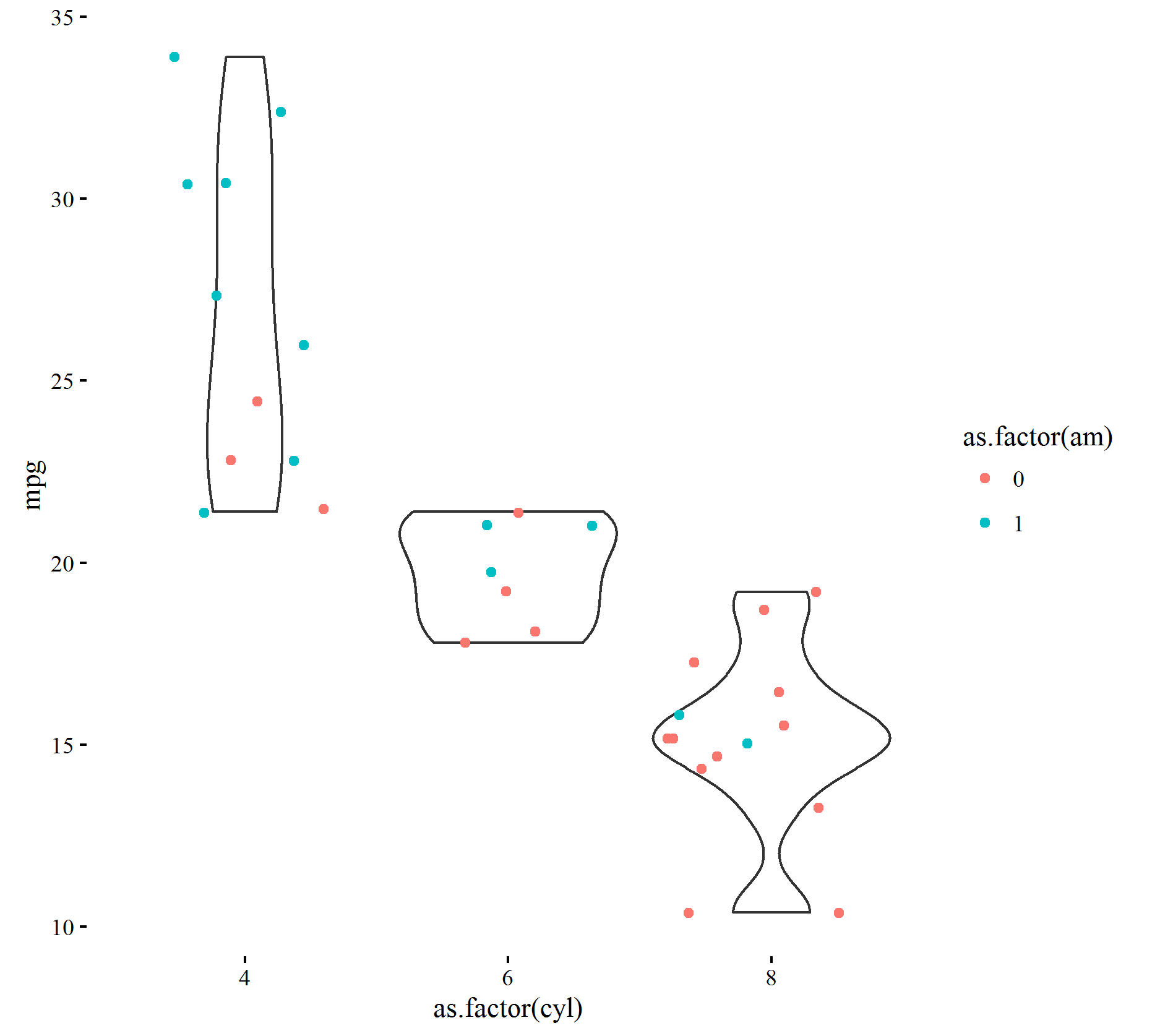So now you know a few graphics and the basics of ggplot use. In the next lesson we will combine dplyr and ggplot to filter, slice and dice through a dataset. Hopefully we will get some insights!